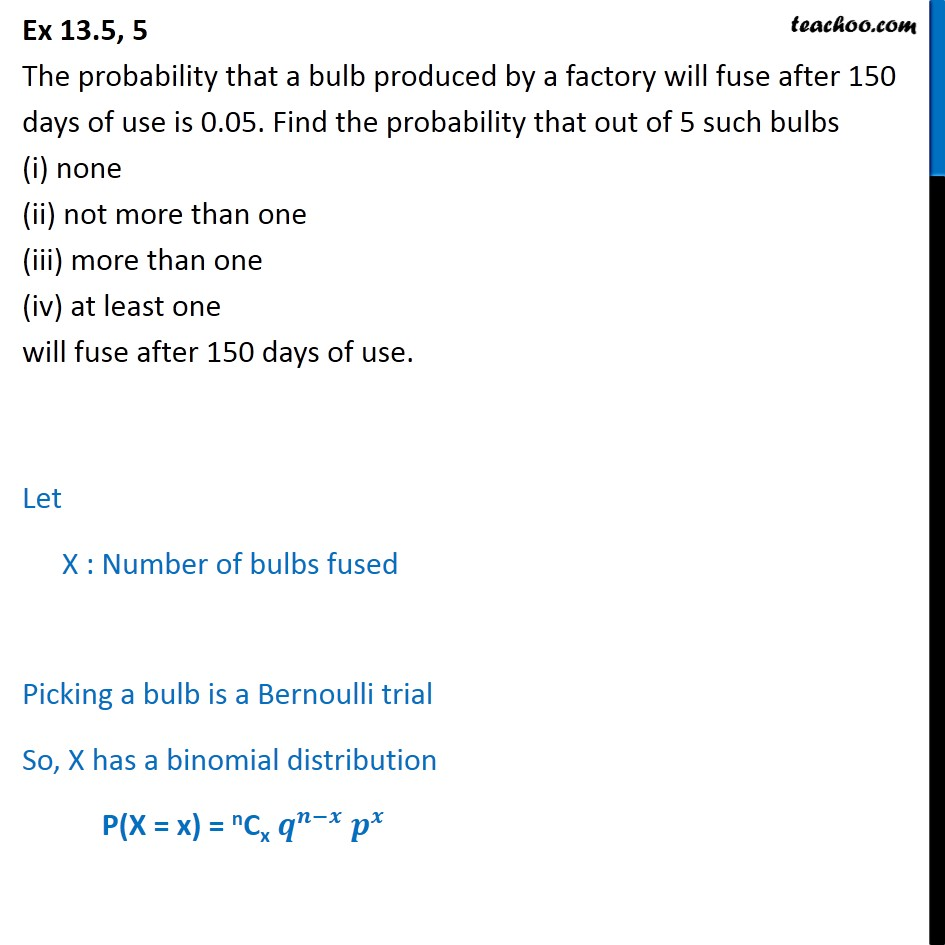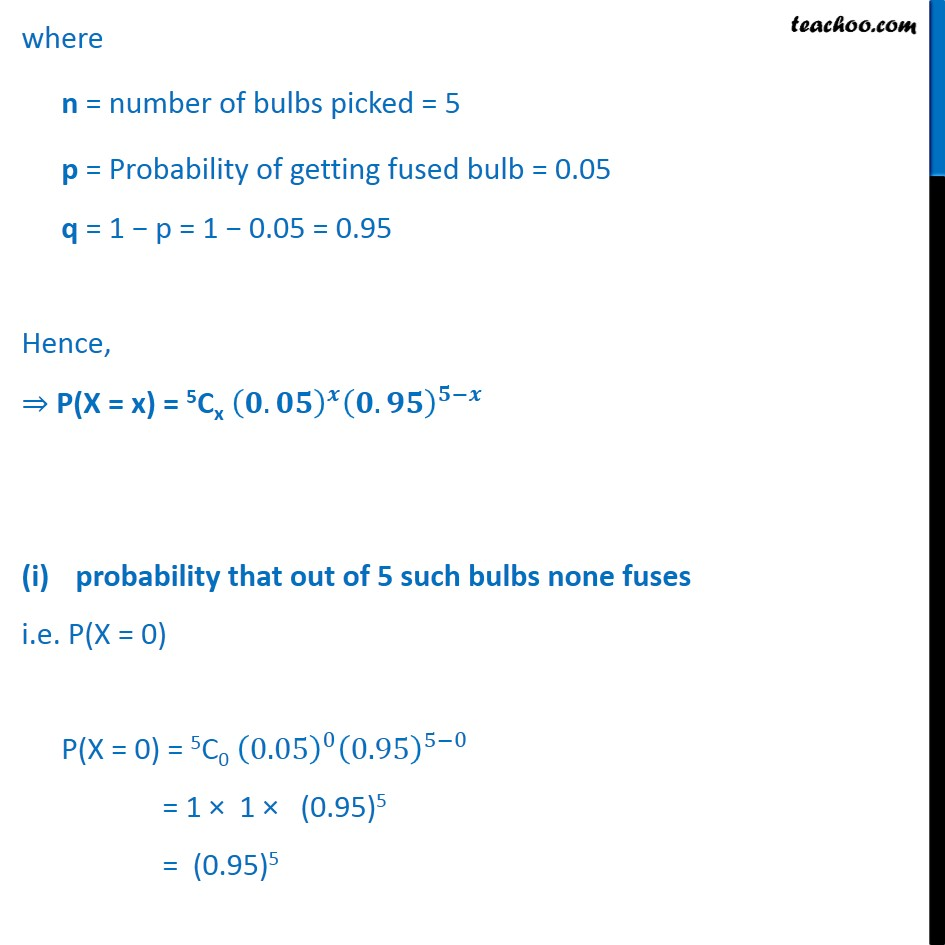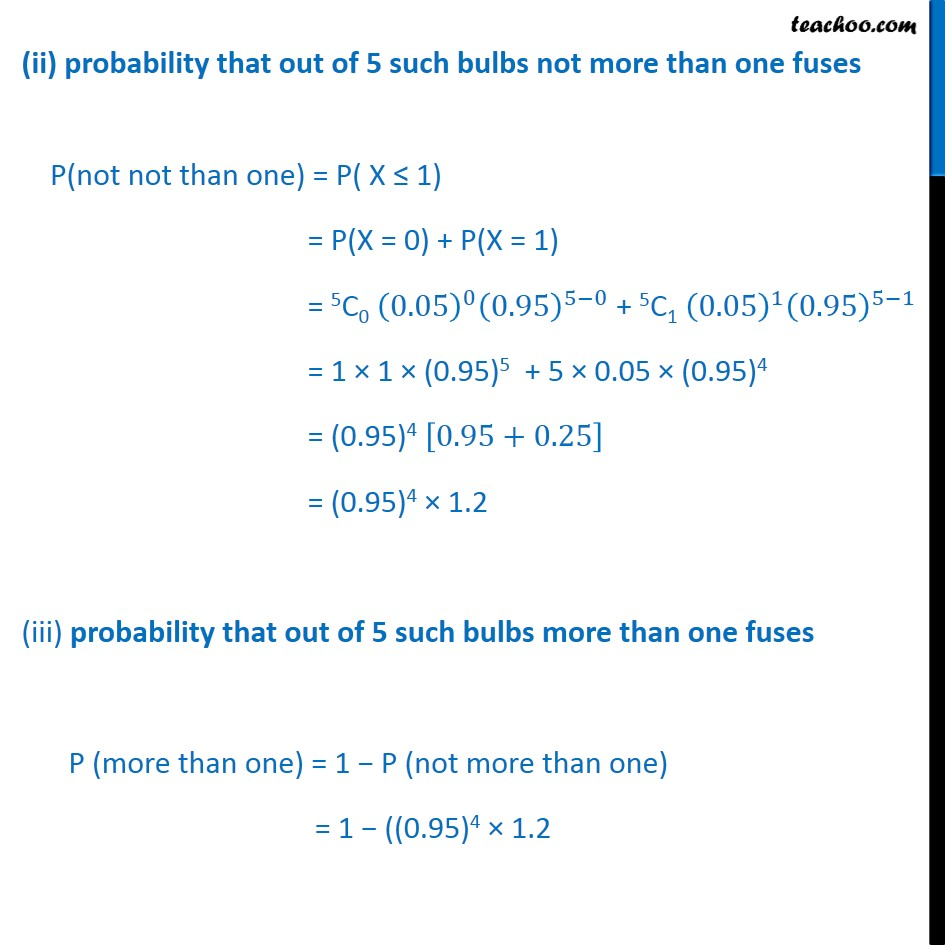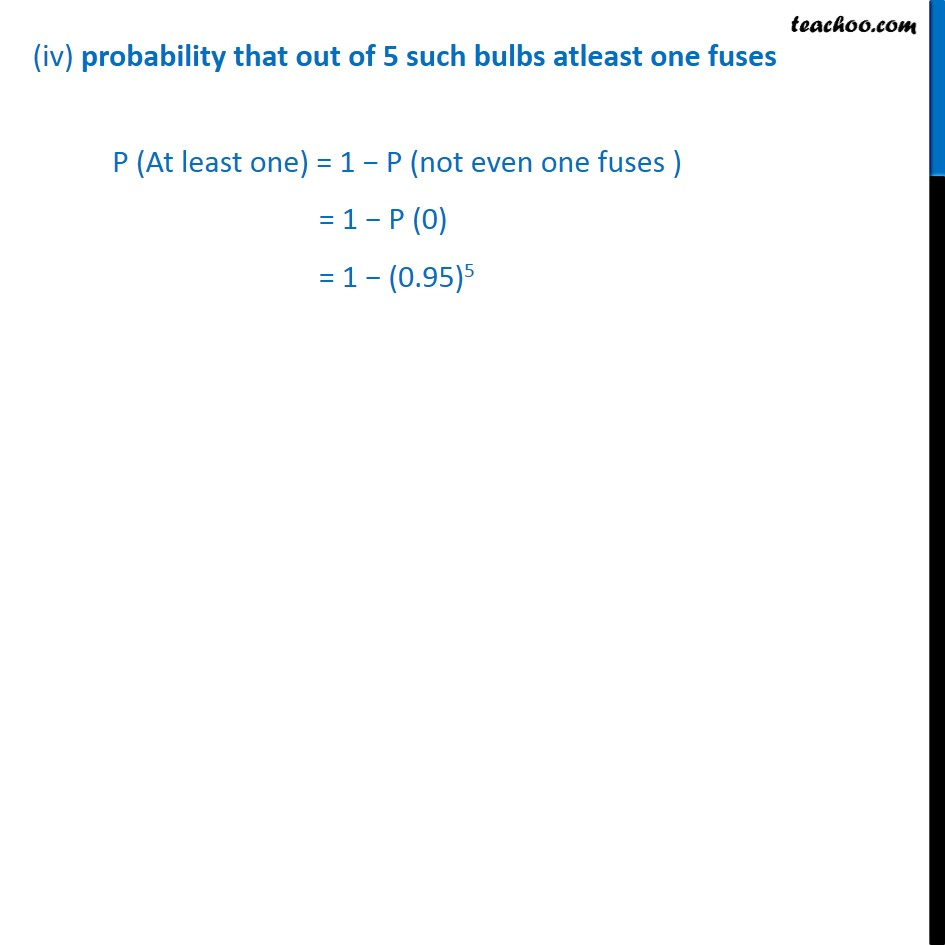1. Chapter 13 Class 12 Probability
2. Serial order wise
3. Ex 13.5

Transcript

Ex 13.5, 5 The probability that a bulb produced by a factory will fuse after 150 days of use is 0.05. Find the probability that out of 5 such bulbs (i) none (ii) not more than one (iii) more than one (iv) at least one will fuse after 150 days of use. Let X : Number of bulbs fused Picking a bulb is a Bernoulli trial So, X has a binomial distribution P(X = x) = nCx where n = number of bulbs picked = 5 p = Probability of getting fused bulb = 0.05 q = 1 p = 1 0.05 = 0.95 Hence, P(X = x) = 5Cx . . probability that out of 5 such bulbs none fuses i.e. P(X = 0) P(X = 0) = 5C0 0.05 0 0.95 5 0 = 1 1 (0.95)5 = (0.95)5 (ii) probability that out of 5 such bulbs not more than one fuses P(not not than one) = P( X 1) = P(X = 0) + P(X = 1) = 5C0 0.05 0 0.95 5 0 + 5C1 0.05 1 0.95 5 1 = 1 1 (0.95)5 + 5 0.05 (0.95)4 = (0.95)4 0.95+0.25 = (0.95)4 1.2 (iii) probability that out of 5 such bulbs more than one fuses P (more than one) = 1 P (not more than one) = 1 ((0.95)4 1.2 (iv) probability that out of 5 such bulbs atleast one fuses P (At least one) = 1 P (not even one fuses ) = 1 P (0) = 1 (0.95)5

Ex 13.5